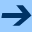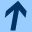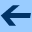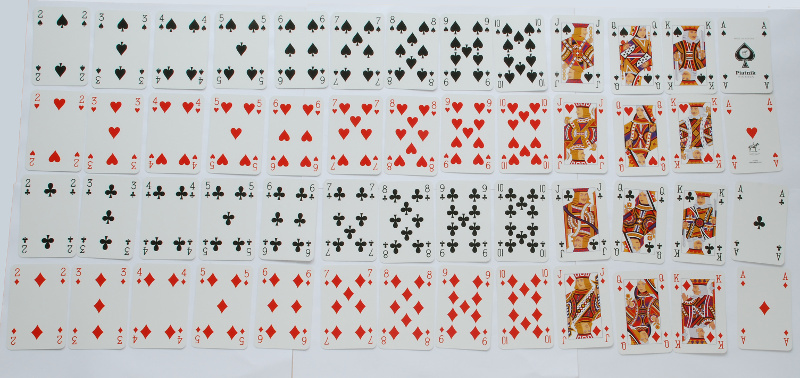Data classes

# Data classes

Objects are the basis of object-oriented programming. In Kotlin, every piece of data is an object. Each object has a type, such as Int, Double, String, Pair, List<Int>, or MutableList<String>. The type of an object determines what you can do with the object. When you use an object, you should think of the object as a black box, and you need not know what really happens inside the object. All you need to know is what the object does, not how it implements its functionality.

A class defines a new type of object. You can also think about a class as a "blueprint" for objects. Once you define a class, you can create objects from the blueprint.

#### Data classes

A common use of classes is to define objects with a number of attributes. For instance:

• A point in the plane has an $$x$$-coordinate and a $$y$$-coordinate. Depending on the application, the coordinates could be integers, or floating-point numbers.
• A date has a year, month, and day.
• A student object has (at least) a name, a student number, and a major.
• A playing card (such as in Blackjack) has a suit (clubs, spades, hearts, or diamonds) and a face value (2,3,…,10, Jack, Queen, King, Ace).Such a simple class is implemented as a data class:

>>> data class Point(val x: Int, val y: Int)

Point represents a two-dimensional point. It has two fields, namely x and y. We can create Point objects as follows:
>>> var p = Point(2, 5)
>>> p
Point(x=2, y=5)

Not that Point(2, 5) looks like a function call, and in fact it is a call to the constructor for the Point class.

Once we have a Point object, we can access its fields using dot-syntax:

>>> p.x
2
>>> p.y
5


We can also print Point objects using println.

>>> println(p)
Point(x=2, y=5)


We can compare two Point objects using == and !=. Two points are equal if and only if all their fields are equal.


>>> val q = Point(7, 19)
>>> val r = Point(2, 5)
>>> p == r
true
>>> p == q
false
>>> p != q
true


Let's now look at the other examples from above: A date object could be defined like this:

>>> data class Date(val year: Int, val month: Int, val day: Int)
>>> val d = Date(2016, 4, 23)
>>> d.month
4
>>> d.day
23

A student object might look like this:
>>> data class Student(val name: String, val id: Int, val dept: String)
>>> val s = Student("Otfried", 13, "CS")
>>> s.id
13

And a Blackjack card object could look like this:
>>> data class Card(val face: String, val suit: String)
>>> val c = Card("Ace", "Diamonds")
>>> c.suit
Diamonds
>>> println(c)
Card(face=Ace, suit=Diamonds)Data classes